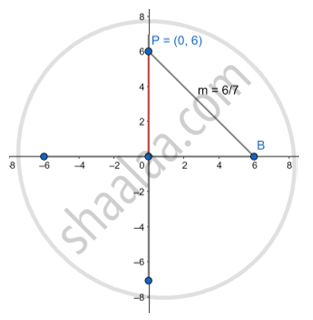# Write the Equation of a Line Passing Through the Point P (0,6) and Having Slope 6/7. - Geometry

Write the equation of a line passing through the point P (0,6) and having slope 6/7.

#### SolutionLet P (0,6) =(x1,y1)

y-y1=m(x-x1)

"y"-6 = 6/7 ("x"-0)

"y"-6 = 6/7"x"

7y-42=6x

Slope-intercept equation ⇒ 6"x-7y"=42

Concept: Slope of a Line
Is there an error in this question or solution?